# Optimization of the Structure of a Solar Air Heater Fitted with V-Shaped Perforated Baffles

Optimization of the Structure of a Solar Air Heater Fitted with V-Shaped Perforated Baffles

Chunlong Zhuang Boheng Fu* Guangqing Huang Hongyu Zhang

Department of National Defense Architecture Planning & Environmental Engineering, LEU, Chongqing 401311, China

Corresponding Author Email:
fbhcy09@163.com
Page:
604-608
|
DOI:
https://doi.org/10.18280/ijht.340408
|
Accepted:
|
Published:
31 December 2016
| Citation

OPEN ACCESS

Abstract:

Our research objective is a solar air heater fitted with V-shaped perforated baffles. Experimental data is used to verify a mathematical model for heat transfer by solar air heater. By calculating fitted values, we obtain the empirical relationship of flow resistance coefficients so as to more accurately predict the performance of the collector. Comprehensive evaluation indices (thermal efficiency and effective efficiency) are employed to analyze the effect of the internal structure of the solar air heater on its performance, and the corresponding structure parameters undergo simulation and optimization. The result shows that the smaller the perforation spacing, plate spacing, and the air layer thickness, the higher the thermal efficiency, but the greater the flow resistance within the collector. Excessively short collector length reduces the thermal efficiency and effective efficiency. When the thermal efficiency and effective efficiency are relatively high, the corresponding parameters are those of the optimal structure of the collector. Thus, the best perforation spacing is 0.006 ~ 0.012 m, the best De /D is 1.4, the optimal plate interval is 0.3 ~ 0.4 m, the air layer depth should be at the range of 0.05 ~ 0.06 m, the solar air heater should be longer than 1.8 m.

Keywords:

V-shaped perforated baffles, Solar air heater, Flow resistance coefficient, Thermal efficiency, Effective efficiency.

1. Introduction

When air flows through the rectangular duct of a traditional flat-plate solar air heater, a viscous laminar flow of great thickness forms along duct wall surface. As a result, heat transfer coefficients between heat absorber and air lower down, which increases absorber temperature and heat loss. In order to enhance the heat transfer efficiency of solar air heaters, a reasonable amount of research has been conducted on improvement of the internal structure of solar air heater  [1,2]. Those researchers attempt to find a modified internal structure that helps intensify air turbulence so as to lift the heat transfer coefficients between heat absorber and air. One of the attempts is to add ribs, baffles or blocks to heat collectors. Momin et al.  experimentally investigated the effect of geometrical parameters of V-shaped ribs, on heat transfer and fluid flow characteristics of a rectangular duct of a solar air heater. Sriromreun  examined the heat transfer and flow friction characteristics in a channel of aspect ratio of 10 fitted with the in-phase and out-phase 45 ° Z-baffles for the Reynolds number from 4,400 to 20,400. Promvonge  studied heat transfer and friction loss behaviors for air flow through a channel fitted with a multiple 60 ° V-baffle turbu-lator. Alam et al.  reported that different turbulence promoters were used in solar air heater and found that blocks placed in the duct can effectively increase the coefficient of heat transfer. Due to its large height, use of such blocks is usually accompanied by high penalty of pressure drop and hot spot.

In order to eliminate these hot spots and reduce pressure drop, attempts have been made by researchers to offset this effect by creating perforations in these blocks. Sara [7,8] reported that solid blocks could lead to energy loss up to 20 % despite significantly enhanced heat transfer due to the increased surface area. The energy loss was recovered by perforations in the blocks so that it was possible to achieve energy gains up to 40 %.These perforated blocks not only reduce pressure drop but also increase heat transfer. Hwang and Liou  investigated heat transfer in a channel with perforated fences and compared the thermal performance of three types of turbulence promoters: solid type, perforated type, and slit type. Karwa et al. [10,11] investigated the heat transfer in rectangular duct equipped with half and fully perforated baffles. Baffles with half perforation corresponding to relative roughness pitch of 7.2 were found to yield best performance. Chamoli and Thakur  investigated the effect of open area ratio and relative hole position of V-shaped perforated baffles placed in the duct of solar air heater. Huang et al.  examined the perforated baffles in a square channel and higher values of heat transfer coefficient are found in case of perforated baffles when compared with solid baffles. Transverse solid and perforated blocks have been investigated to study the effect of hole diameter, open area ratio, number of blocks, hole inclination and different shapes on heat transfer [7,8,14,15].

However, these analyses separate the heat transfer characteristics from the resistance characteristics of solar air heaters with perforated blocks. Due to the limitation of the structure parameters during the experiment, the previous literatures lack specific optimization of the perforation spacing, pore type, baffle interval, air layer thickness and collector length. For the solar air heater fitted with V-shaped perforated baffles, we let the baffle be as high as air layer. This design can enhance heat transfer at the same time when the baffles provide support to transparent cover plates so that reducing the impact force acted on them. De /D is also used to show different perforation shapes. We calculate fitted values using experimental data, and acquired the empirical relationship of flow resistance coefficients. Accordingly, we conduct non-isothermal treatment of the heat collecting part,  establish a mathematical model for heat transfer of air heating components, and verify its effectiveness. Besides, we adopt the numerical simulation method to combine the heat transfer characteristics and the resistance characteristics. Comprehensive evaluation indices (thermal efficiency and effective efficiency) are employed to analyze the effect of the internal structure of the solar air heater on its thermal performance, and the corresponding structure parameters undergo simulation and optimization.

2. The Collector Structure

Figure 1 shows the solar air heater fitted with V-shaped perforated baffles. Jets through perforations strengthen the effect of convective heat transfer between air flow and absorber plate, rendering air turbulence and mixture more and air flow in dead zone less.

The material of transparent cover plates is 3 mm ordinary glasses with the transmittance of 82 % and the thermal conductivity of 0.75 W/(m·K). The absorber plate is made of 1mm steel plate, 600 mm×1500 mm. On the outside surface of the absorber plate is black coating, whose absorptivity is 0.95 and emissivity is 0.77. The air layer is 60 mm thick. 6 mm diameter poles are punched through the baffles. The included angle between baffle and structure wall is 60 °.

## 1.pngFigure 1. The collector structure

3. Mathematical Model

3.1 Simplification of the mathematical model

Figure 2 shows the air heater model and the corresponding network partition.

## 2a.png(a) Physical model

## 2b.png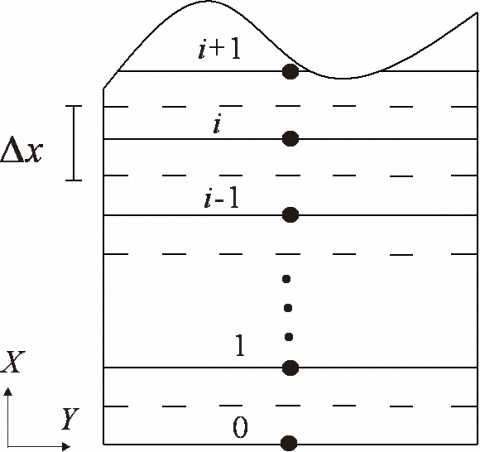(b) Grid drawing

Figure 2. The air heater model and grid drawing

In order to ensure that the model is accurate and convenient to find solutions, we propose the following assumptions.

(1) Thermal transfer is a steady process.

(2) Heat transfer of the transparent cover plate and the absorber plate along axis Y and axis Z is negligible. Heat transfer at the backside and the lateral side of the air heater is negligible.

(3) The coefficient of convective heat transfer is calculated by the mean flow velocity in the corresponding space.

(4) Heat conduction of the transparent cover plate and the absorber plate on the area boundary is negligible.

3.2 Establishment of the mathematical model

Based on thermal equilibrium method, we build up a control equation set of the transparent cover plate, the absorber plate, and air flow in the channel. By using the finite difference technique, we divide the air heater into i=0, 1, 2,..., n controllers along the direction of X, in an attempt to find the solution to air temperature at the outlet of the air heater.

3.2.1 The energy equilibrium equation of node i for the transparent cover plate

For the cover plate fraction in region 1:

$\alpha_{\mathrm{g}} I_{\mathrm{c}}+h_{1}\left[T_{\mathrm{a}}-T_{\mathrm{gl}}(i)\right]$$+h_{2}\left[T_{\mathrm{f} 1}(i)-T_{\mathrm{gl}}(i)\right]+h_{3}\left[T_{\mathrm{bl}}(i)-T_{\mathrm{gl}}(i)\right]$$+\lambda_{\mathrm{g}} \delta_{\mathrm{g}} \frac{T_{\mathrm{gl}}(i-1)-2 T_{\mathrm{gl}}(i)+T_{\mathrm{gl}}(i+1)}{\Delta x^{2}}-q_{\mathrm{radi}}=0$(1)

$q_{\mathrm{rad} 1}=\varepsilon_{\mathrm{g}} \sigma_{\mathrm{b}}\left(T_{\mathrm{g} 1}(i)^{4}-F_{\mathrm{gs}} T_{\mathrm{sky}}^{4}-F_{\mathrm{gg}} T_{\mathrm{grad}}^{4}\right)$(2)

For the cover plate fraction in region 2:

$\alpha_{\mathrm{g}} I_{\mathrm{c}}+h_{1}\left[T_{\mathrm{a}}-T_{\mathrm{g} 2}(i)\right]$$+h_{2}\left[T_{\mathrm{f} 2}(i)-T_{\mathrm{g} 2}(i)\right]+h_{3}\left[T_{\mathrm{b} 2}(i)-T_{\mathrm{g} 2}(i)\right]$$+\lambda_{\mathrm{g}} \delta_{\mathrm{g}} \frac{T_{\mathrm{g} 2}(i-1)-2 T_{\mathrm{g} 2}(i)+T_{\mathrm{g} 2}(i+1)}{\Delta x^{2}}-q_{\mathrm{rad} 2}=0$(3)

$q_{\mathrm{rad} 2}=\varepsilon_{\mathrm{g}} \sigma_{\mathrm{b}}\left(T_{\mathrm{g} 2}(i)^{4}-F_{\mathrm{gs}} T_{\mathrm{sky}}^{4}-F_{\mathrm{gg}} T_{\mathrm{grad}}^{4}\right)$(4)

3.2.2 The energy equilibrium equation of node i for the absorber plate

For the absorber plate fraction in region 1:

$\tau_{\mathrm{g}} \alpha I_{\mathrm{c}}+h_{2}\left[T_{\mathrm{f} 1}(i)-T_{\mathrm{bl}}(i)\right]+h_{3}\left[T_{\mathrm{gl}}(i)-T_{\mathrm{bl}}(i)\right]$$+\lambda_{\mathrm{b}} \delta_{\mathrm{b}} \frac{T_{\mathrm{bl}}(i-1)-2 T_{\mathrm{b} 1}(i)+T_{\mathrm{b} 1}(i+1)}{\Delta x^{2}}=0(5) For the absorber plate fraction in region 2: \tau_{\mathrm{g}} \alpha I_{\mathrm{c}}+h_{2}\left[T_{\mathrm{f} 2}(i)-T_{\mathrm{b} 2}(i)\right]+h_{3}\left[T_{\mathrm{g} 2}(i)-T_{\mathrm{b} 2}(i)\right]$$+\lambda_{\mathrm{b}} \delta_{\mathrm{b}} \frac{T_{\mathrm{b} 2}(i-1)-2 T_{\mathrm{b} 2}(i)+T_{\mathrm{b} 2}(i+1)}{\Delta x^{2}}=0$(6)

Baffles and the absorber plate have equal coefficients of heat conduction and heat storage, because they are made of the same material. Therefore, we suppose

$T_{\mathrm{k}}(i)=T_{\mathrm{b} 2}(i)$(7)

3.2.3 The energy equilibrium equation of node i for air flow in the channel

For air flow in region 1:

$\lambda d \frac{T_{\mathrm{f} 1}(i-1)-2 T_{\mathrm{f} 1}(i)+T_{\mathrm{f} 1}(i+1)}{\Delta x^{2}}+h_{2}\left[T_{\mathrm{gl}}(i)-T_{\mathrm{f} 1}(i)\right]$$+h_{2}\left[T_{\mathrm{bl}}(i)-T_{\mathrm{f} 1}(i)\right]+\frac{h_{2} e(1-p)}{W \cos \theta}\left[T_{\mathrm{k}}(i)-T_{\mathrm{f} 1}(i)\right]$$+\frac{h_{\mathrm{hole}} 2 e \delta_{\mathrm{k}} p}{R \cos \theta}\left[T_{\mathrm{k}}(i)-T_{\mathrm{f} 2}(i)\right]=$$\rho c_{\mathrm{p}} \frac{Q_{\mathrm{f} 1}(i) T_{\mathrm{f} 1}(i)-Q_{\mathrm{fl}}(i-1) T_{\mathrm{f} 1}(i-1)-Q T_{\mathrm{f} 2}(i-1) / 2 n}{3600 W \Delta x}(8) For air flow in region 2: \lambda d \frac{T_{\mathrm{f} 2}(i-1)-2 T_{\mathrm{f} 2}(i)+T_{\mathrm{f} 2}(i+1)}{\Delta x^{2}}$$+h_{2}\left[T_{\mathrm{g} 2}(i)-T_{\mathrm{f} 2}(i)\right]$$+h_{2}\left[T_{\mathrm{b} 2}(i)-T_{\mathrm{f} 2}(i)\right]+\frac{h_{2} e(1-p)}{W \cos \theta}\left[T_{\mathrm{k}}(i)-T_{\mathrm{f} 2}(i)\right]$$-\rho c_{\mathrm{p}} \frac{Q_{\mathrm{f} 2}(i) T_{\mathrm{f} 2}(i)-Q_{\mathrm{f} 2}(i) T_{\mathrm{f} 2}(i-1)}{3600 W \Delta x}=0$(9)

3.2.4 The energy equilibrium equation of node i for non-baffle sections

With the use of the said energy equilibrium equation (1)-(6), we obtain the energy equilibrium equation of air flow in the duct:

$\lambda d \frac{T_{\mathrm{f}}(i-1)-2 T_{\mathrm{f}}(i)+T_{\mathrm{f}}(i+1)}{\Delta x^{2}}+h_{2}\left[T_{\mathrm{g}}(i)-T_{\mathrm{f}}(i)\right]$$+h_{2}\left[T_{\mathrm{b}}(i)-T_{\mathrm{f}}(i)\right]-\rho c_{\mathrm{p}} \frac{Q T_{\mathrm{f}}(i)-Q T_{\mathrm{f}}(i-1)}{2 \times 3600 \mathrm{W} \Delta x}=0(10) where h2 is calculated by the model proposed by L. M. Augustus et al. , hhole by the C. F. Kutscher model . h_{1}=5.7+3.8 v_{w}(11) h_{2}=\frac{N u \times \lambda}{H}=\frac{0.664 \lambda \cdot \mathrm{Re}^{0.5} \cdot \mathrm{Pr}^{0.333}}{H}$$=\frac{0.664 \lambda\left(\frac{v_{\mathrm{f}} H}{v}\right)^{0.5} \cdot \operatorname{Pr}^{0.333}}{H}$(12)

$h_{3}=\sigma_{\mathrm{b}}\left(\overline{T_{\mathrm{g}}}^{2}+\overline{T_{\mathrm{b}}}^{2}\right)\left(\overline{T_{\mathrm{g}}}+\overline{T_{\mathrm{b}}}\right) /\left(\frac{1}{\varepsilon_{\mathrm{g}}}+\frac{1}{\varepsilon_{\mathrm{b}}}-1\right)$(13)

$h_{\mathrm{hole}}=\frac{N u_{\mathrm{hole}} \times \lambda}{D \mathrm{e}}=$$\frac{2.75 \lambda\left[\left(\frac{B}{D \mathrm{e}}\right)^{-1.21} \mathrm{Re}^{0.43}+0.011 p \operatorname{Re}\left(\frac{v_{\mathrm{f} 2}}{v_{\mathrm{app}}}\right)^{0.48}\right]}{D \mathrm{e}}$(14)

Boundary conditions:

Tf1 (0)=Tf2 (0)=Tin, Tb1 (0)=Tb2 (0)=Tb, Tg1 (0)=Tg2 (0)=Tg,

Qf1(0)=Qf2(n)=0, Qf2(0)=Qf1(n)=Q/2

In addition, adiabatic boundary conditions are applied to the upper boundary and lower boundary of the rest of the air heater.

Matlab is used to calculate air temperature in the outlet. The corresponding steps are

(1) Input in matlab environmental parameters, air heater structure parameters, boundary conditions, and the number of nodes n. The step length is set as B ∙ cosθ.

(2) The average temperature of the transparent cover plate and the absorber plate is assumed as ($\overline{T_{\mathrm{g}}}, \overline{T_{\mathrm{b}}}$).

(3) Judge whether both of the differences between mean air temperature and the assumed values are smaller than the iteration precision a, which is taken as 10-4. If it is, the calculation result has converged, or otherwise shows no sign of convergence. The calculated values displace the previous assumed values and continue to be iterated, until they satisfy the conditions of convergence.

4. Model Verification and Flow Resistan- Ce Coefficient Fitting

4.1 Model verification

Total radiation table TRT-2A is used to record radiation strength (measure scope: 0~2000 W/m2, sensitivity: 10.119×10-6 V/(W∙m2)). It is placed at the side of the solar air heater, with the sensing surface parallel to the absorber plate. We install two T-shaped thermocouples at the air inlet ant outlet to test air temperature. TSI air velocity tester is used to automatically read in the air velocity and pressure drop at the air inlet and outlet (measure scope: 0~78.7 m/s, -3735 ~ +3735 Pa, precision: ±1.5 %, ±1 %).

The test lasts 7 days, during which we undertake model verification on one sunny day. The air supply volume is 100 m3/h. Figure 3 shows the daily radiation strength, the measured value of air temperature at the air inlet, as well as the simulated value and measured value of air temperature at the air outlet. The air outlet temperature increases first and then decreases as the radiation strength changes.

## 3.png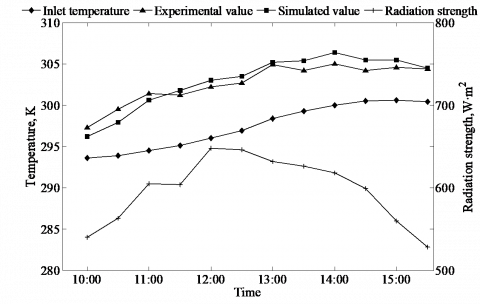Figure 3. Radiation strength and air temperature change at the air inlet and outlet

The mean deviation is used to compare the experimental air temperature and the simulated one at the air outlet:

$\sigma_{\mathrm{p}}=\frac{1}{n} \sum_{n}\left|T_{\mathrm{out}, \mathrm{s}}-T_{\mathrm{out}, \mathrm{m}}\right|$(15)

The simulated and experimental outlet air temperatures have achieved good agreement with the same trend. The calculated mean deviation is 1.05 K, and relative mean deviation is 0.35 %. This is caused by delay in measured temperature and uneven heat transfer due to the unevenness of actual air flow field in the internal structure of the air heater. As a whole, the measured value and the simulated value fit well with each other.

4.2 Air flow resistance coefficient fitting

The equation of flow resistance when air flows through the air heater is given as

$\Delta P_{\mathrm{f}}=f \frac{H}{D \mathrm{e}} \frac{4 \rho v_{\mathrm{f}}^{2}}{2}$(16)

The experimental data of inlet-outlet pressure drops changing with the change of air flow volume, porosity, baffle interval, and De /D are used for fitting, and a corresponding empirical relation of flow resistance coefficient is obtained. De is the equivalent diameter of different pore shapes, D represents the equivalent diameter of round holes. When the perforation area remains unchanged, different values of De /D reflect different pore types.

## 4.png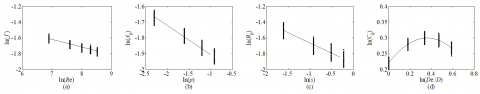Figure 4. Experimental data and fitting

We let $f=A_{0} \operatorname{Re}^{n_{1}}, \ln (f)=\ln \left(A_{0}\right)+n_{1} \ln (\mathrm{Re})$

According to Figure 4(a), the fitting value is given below:

$f=A_{0} \mathrm{Re}^{-0.092}$(17)

Similarly, we let $\ln \left(A_{0}\right)=\ln \left(B_{0}\right)+n_{2} \ln (p)$

According to Figure 4(b), the fitting value is given below:

$A_{0}=B_{0} p^{-0.193}$(18)

We let $\ln \left(C_{0}\right)=\ln \left(D_{0}\right)+D_{1} \ln (\theta)+D_{2}[\ln (\theta)]^{2}$

According to Figure 4(c), the fitting value is given below:

$B_{0}=C_{0} s^{-0.258}$(19)

We let $\ln \left(C_{0}\right)=\ln \left(D_{0}\right)+D_{1} \ln (\theta)+D_{2}[\ln (\theta)]^{2}$

According to Figure 4(d), the fitting value is given below:

$C_{0}=0.2176 \cdot(D e / D)^{0.453} \exp \left\{-0.624[\ln (D e / D)]^{2}\right\}$(20)

We obtain the final fitting value which is given below:

$f=0.2176 \cdot \mathrm{Re}^{-0.092} p^{-0.193} s^{-0.258}(D e / D)^{0.453}$

$\cdot \exp \left\{-0.624[\ln (D e / D)]^{2}\right\}$(21)

5. Result and Analysis

We take into account the effect of internal air flow resistance and the energy loss of draught fan on air heater performance. Thermal efficiency and effective efficiency  are used as the objective functions in this paper. We analyze what one factor impacts on heater performance while all other factors are equal.

$\eta_{r h}=\frac{Q_{\mathrm{u}}-\left(W_{\mathrm{p}} / C_{\mathrm{f}}\right)}{I_{\mathrm{c}} A}=\eta-\frac{Q \Delta P_{\mathrm{f}} /\left(\eta_{\mathrm{pm}} C_{\mathrm{f}}\right)}{3600 I_{\mathrm{c}} A}$(22)

We let Ic=500 W/m2, Q=100 m3/h, Tin=293 K, Tb=305 K, Tg=292 K, vw=0.3 m/s, Ta=283 K, s=0.4 m, p=12 %, θ=60 °, e=0.05 m. Other parameters are set the same as those in Section 1.

5.1 Effects of perforation spacing

## 5.png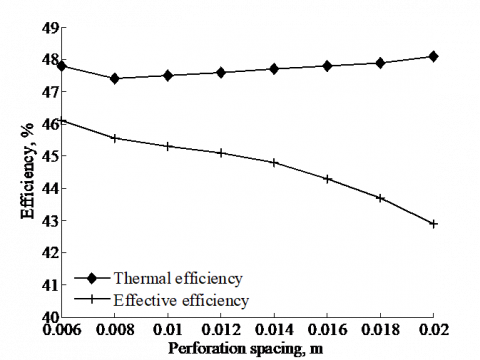Figure 5. Effects of perforation spacing on air heater performance

Figure 5 displays the change of air heater performance when perforation spacing is enlarged from 0.006 m to 0.020 m, ceteris paribus. When perforation spacing increases from 0.006 m to 0.008 m, the turbulence area shrinks, and the thermal efficiency drops from 47.8 % to 47.4 %. When perforation spacing continues rising up to 0.020 m, air flow speed through perforations amplifies. During this period, impact jet dominates, and the convection heat transfer coefficient arises. The thermal efficiency witnesses an increase from 47.4 % to 48.1 %. However, as perforation spacing is further enlarged, air flow resistance rockets, which brings the constant reduction in effective efficiency from 46.1 % to 42.9 %. Therefore, after overall consideration, we suggest the ideal perforation spacing be controlled within the range of 0.006 ~ 0.012 m, because the thermal efficiency and effective efficiency are relatively high when the perforation spacing is 0.006 ~ 0.012 m and the effective efficiency reduces rapidly when the spacing is above 0.012 m.

5.2 Effects of pore shapes

## 6.png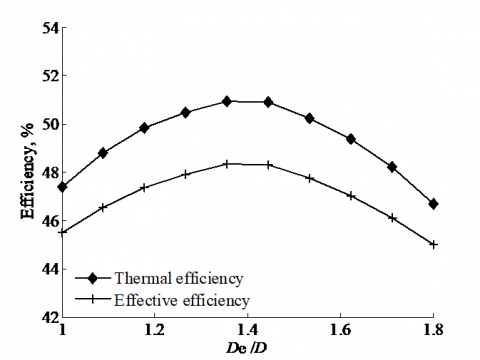Figure 6. Effects of De /D on air heater performance

Figure 6 displays the change of air heater performance when De /D increases from 1 to 1.8, ceteris paribus. As can be seen from Figure 6, thermal efficiency first rises from 47.4 % to 51.1 %, and then lowers down to 46.7 %. This phenome-non is attributed to this: when De /D increases to 1.4, the transverse pore length increases, which encourages transverse air flow to blend as a spur to the slight rise in thermal efficiency; however, when De /D continue rising up, the mixture of longitudinal air flow declines, and thus thermal efficiency finds a descending trend. According to Equation (21), as De /D inclines, air flow resistance coefficients increases first and then decreases, and the effective efficiency rises from 45.5 % to 48.4 %, followed by a decline to 45.0 %. The reason is that in comparison to round pores, square pores with its sharp angle will locally affect air flow and result from an increase of flow resistance. Therefore, after overall consideration, we suggest the ideal De /D value be 1.4,  because the thermal efficiency and effective efficiency reach the maximum when De /D equals 1.4.

5.3 Effects of baffle intervals on air heater performance

## 7.png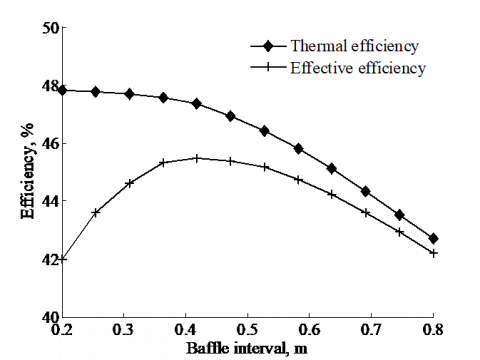Figure 7. Effects of baffle intervals on air heater performance

Figure 7 displays the change of air heater performance when baffle interval is enlarged from 0.2 m to 0.8 m, ceteris paribus. When baffle interval increases, the number of baffles is reduced, which weakens the effect of convective heat transfer and accordingly lowers thermal efficiency from 47.8 % to 42.7 %. We consider the impact of air flow resistance that smaller baffle intervals corresponds to greater resistance, which explains the phenomenon that effective efficiency first increases from 42.0 % to 45.5 % and then drops down to 42.2 %. Therefore, on the whole, we suggest the ideal baffle interval be controlled within the range of 0.3 ~ 0.4 m, because the thermal efficiency is relatively high when the baffle interval is 0.2 ~ 0.4 m and the effectively efficiency is relatively high when the interval is 0.3 ~ 0.5 m.

5.4 Effects of air layer thickness on air heater performance

Figure 8 displays the change of air heater performance when air layer thickness is enlarged from 0.03 m to 0.12 m, ceteris paribus. As air layer becomes thicker, thermal efficiency is reduced from 46.4 % to 43.4 %. Thicker air layer means lower cross-section flow velocity and a smaller heat transfer coefficient. Thinner air layer can speed up air flow, but may be accompanied by insufficient heat transfer. As a result, when air layer is thickened from 0.03 m to 0.06 m, the thermal efficiency witnesses a tendency of relatively low decline. Also, as air layer becomes thinner, air flow resistance strengthens, and thus effective efficiency first increases from 42.3 % to 45.5 % and then drops down to 42.7 %. Therefore, after overall consideration,  we suggest the ideal air layer thickness be controlled within the range of 0.05 ~ 0.06 m, because the thermal efficiency and effective efficiency are relatively high when the air layer thickness is 0.05 ~ 0.06 m.

## 8.png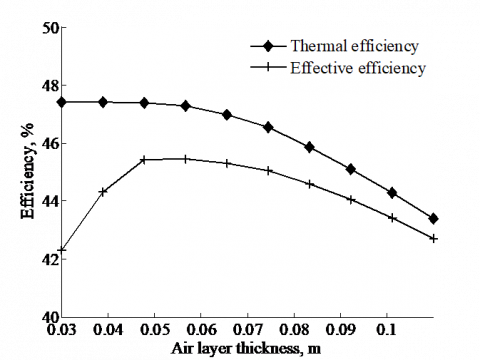Figure 8. Effects of air layer thickness on air heater performance

5.5 Effects of air heater length on air heater performance

## 9.png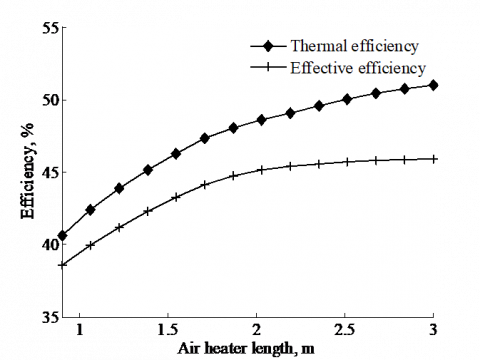Figure 9. Effects of air heater length on air heater performance

Figure 9 displays the change of air heater performance when air heater length is broadened from 0.9 m to 3.0 m, ceteris paribus. As air heater becomes longer, thermal efficiency rises from 40.6 % to 51.2 %. The main reason is that: too short air heater restricts the number of baffles, and thus the turbulence effect is weakened before thermal efficiency declines. When the air heater length increases from 2 m to 3 m, the total solar radiation volume collected by the air heater amplifies, which is the direct cause to the gradually decreasing temperature difference between air flow in the rear of the air heater and the absorber plate. As a result, the heat exchange effect lessens, and thermal efficiency growth rate decreases. Meanwhile, as air heater becomes longer, the number of perforations increase, which intensifies the flow resistance. When the air heater length increases from 1.8 m to 3 m, the growth rate of effective efficiency is relatively low. Therefore, after overall consideration, we suggest the ideal air heater length be larger than 1.8  m, because when the length of the collector is less than 1.8 m, the performance of the collector is greatly affected as showcased by the relatively low thermal efficiency and efficiency.

6. Conclusions

Through experimental research and theoretical analysis on the solar air heater equipped with V-shaped perforated blocks, we obtain the following conclusions:

(1) After comparing the simulated temperature and experimental temperature at the air inlet and outlet, we find that their trend is the same, the mean deviation is 1.05 K, and the relative mean deviation is 0.35 %. This means that the established mathematical model is reliable such that it can be based for related theoretical research.

(2) The best perforation spacing is 0.006 ~ 0.012 m, the ideal De /D is 1.4, the optimal plate interval is 0.3 ~ 0.4 m, the air layer depth should be at the range of 0.05 ~ 0.06 m, the solar air heater should be longer than 1.8 m.

Nomenclature
 T temperature, K Tsky effective sky temperature, K Ic solar radiation temperature, W/m2 qrad radiation heat transfer between glass and environment, W/m2 Q air supply volume, m3/h h1 convection heat transfer coefficient between glass and the environment, W/(m2∙K) h2 convection heat transfer coefficient between air flow and absorber plate,  and between air flow and cover plate, W/(m2∙K) h3 radiation heat transfer coefficient between glass and absorber plate, W/(m2∙K) hhole convection heat transfer coefficient when air flows through perforations, W/(m2∙K) d air layer thickness, m e height of baffle, m vf cross-section air flow velocity in the duct, m/s vapp head-on air flow speed against the absorber plate, m/s vw outdoor wind speed, m/s p aperture ratio s baffle interval, m D Dk equivalent diameters of the duct and perforations, respectively, m B center distance between adjacent pores, m W semi-width of air heater, m H baffle sections air heater length, m Re, Nu, Pr Reynolds number, Nusselt number, and Planck number Fgs Fgg the angular coefficient between glass and sky, and between glass and the ground ΔPf air flow resistance in the air heater, Pa f air flow resistance coefficient Cf thermal-mechanical energy conversion factor, 0.02 Wp drought fan power consumption, W Qu effective energy of the air heater, W Greek symbols αg absorptivity of glass cover plate α absorptivity of absorber plate τ transmissivity of glass cover plate λ heat conduction coefficients, W/(m∙K) ε emissivity on the surface, W/(m∙K) σb Boltzmann constant δ thickness, m θ included angle between baffle and heater wall, ° ηrh effective efficiency η thermal efficiency Subscripts a ambient b absorber plate g glass cover plate f flow k baffle gnd ground in inlet out outlet m simulated s experimental 1, 2 region 1, region 2
References

 A. Labed, N. Moummi, A. Benchabane, M Zellouf, “Experimental analysis of heat transfer in the flow channel duct of solar air heaters (SAHs),” Int J Heat & Tech, vol. 33, no. 3, pp. 97-102, Sep., 2015. DOI: 10.18280/ijht.330314.

 S. Bhattacharyya, H. Chattopadhyay, S. Bandyopadhyay, S. Roy, A. Pal, S. Bhattacharjee, “Experimental investigation on heat transfer enhancement by swirl generators in a solar air heater duct,” Int J Heat & Tech, vol. 34, no. 2, pp. 191-196, Jun., 2016. DOI: 10.18280/ijht.340206.

 A.M.E. Momin, J.S. Saini, S.C. Solanki, “Heat transfer and friction in solar air heater duct with V-shaped rib roughness on absorber plate,” Int J Heat Mass Transf, vol. 45, no. 16, pp. 3383-3396, Jul., 2002. DOI: 10.1016/S0017-9310(02)00046-7.

 P. Sriromreun, C. Thianpong, P. Promvonge, “Experimental and numerical study on heat transfer enhancement in a channel with Z-shaped baffles,” Int Commun Heat Mass Transf, vol. 39, no. 7, pp. 945-952, Aug., 2012. DOI: 10.1016/j.icheatmasstransfer.2012.05. 016.

 P. Promvonge, “Heat transfer and pressure drop in a channel with multiple 60° V-baffles,” Int Commun Heat Mass Transf, vol. 37, no. 7, pp. 835-840, Aug., 2010. DOI: 10.1016/j.icheatmasstransfer.2010.04.003.

 T. Alam, R.P. Saini , J.S. Saini, “Heat and flow characteristics of air heater ducts provided with turbulators—a review,” Renew Sustain Energy Rev, vol. 31, no. 31, pp. 289-304, Mar., 2014. DOI: 10.1016/j. rser.2013.11.050.

 O.N. Sara, T. Pekdemir, S. Yapici , M. Yilmaz, “Heat-transfer enhancement in a channel flow with perforated rectangular blocks,” Heat Fluid Flow, vol. 22, no. 5, pp. 509-518, Oct., 2001. DOI: 10.1016/S0142-727X(01)001 17-5.

 O.N. Sara, T. Pekdemir, H. Ersahan, “Thermal performance analysis for solid and perforated blocks on a flat surface in a duct flow,” Energy Convers Manage, vol. 41, no. 10, pp. 1019-1028, Jul., 2000. DOI: 10.1016/S0196-8904(99)00163-6.

 J.J. Hwang ,T.M. Liou, “Heat transfer in a rectangular channel with perforated turbulence promoters using holographic interferometry measurement,” Int J Heat Mass Transf, vol. 38, no. 17, pp. 3197-3207, Nov., 1995. DOI: 10.1016/0017-9310(95)00065-H.

 R. Karwa, B.K. Maheshwari, “Heat transfer and friction in an asymmetrically heated rectangular duct with half and fully perforated baffles at different pitches,” Int Commun Heat Mass Transf, vol. 36, no. 3, pp. 264-268, Mar., 2009. DOI: 10.1016/j.icheatmasstransfer.2008.11. 005.

 R. Karwa, B.K. Maheshwari, N. Karwa, “Experimental study of heat transfer enhancement in an asymmetrically heated rectangular duct with perforated baffles,” Int Commun Heat Mass Transf, vol. 32, no. 1-2, pp. 275-284, Jan., 2005. DOI: 10.1016/j.icheatmasstransfer.2004. 10.002.

 S. Chamoli, N.S. Thakur, “Heat transfer enhancement in solar air heater with V-shaped perforated baffles,” J Renew Sustain Energy, vol. 5, no. 2, pp. 347-355, Mar., 2013. DOI: 10.1063/1.4798411.

 K.D. Huang, S.C. Tzeng, T.M. Jeng, J.R. Wang, S.Y. Cheng, K.T. Tseng, “Experimental study of fluid flow and heat transfer characteristics in the square channel with a perforation baffle,” Int Commun Heat Mass Transf, vol. 35, no. 9, pp. 1106-1112, Nov., 2008. DOI: 10.1016/j.icheatmasstransfer.2008.07.013.

 O.N. Sara, T. Pekdemir, S. Yapmcm, M. Ymlmaz, “ Enhancement of heat transfer from a flat surface in a channel flow by attachment of rectangular blocks,” Int J Energy Res, vol. 25, no. 7, pp. 563-576, Jun., 2001. DOI: 10.1002/er.703.

 S. Shin, J.S. Kwak, “Effect of hole shape on the heat transfer in a rectangular duct with perforated blockage walls,” J Mech Sci Technol, vol. 22, no. 10, pp. 1945-1951, Oct., 2008. DOI: 10.1007/s12206-008-0736-7.

 L.M. Augustus, S. Kumar, “Mathematical modelling and thermal performance analysis of unglazed transpired solar collectors,” Solar Energy, vol. 81, no. 1, pp. 62-75, Jan., 2007. DOI: 10.1016/j.solener.2006.06. 017.

 C.F. Kutscher, “Heat exchanger effectiveness and pressure drop for air flow through perforated plates, with and without crosswind,” ASME Journal of Heat Transfer, vol. 116, no. 2, pp. 391-399, May, 1994. DOI: 10.1115/1.2911411.

 M.K. Gupta, S.C. Kaushik, “Performance evaluation of solar air heater for various artificial roughness geometries based on energy, effective and exergy efficiencies,” Renewable Energy, vol. 34, no. 3, pp. 465-476, Mar. 2009. DOI: 10.1016/j.renene.2008.06. 001.